# Forecasting Methods

Four methods of forecasting revenues

## Top Four Types of Forecasting Methods

There are four main types of forecasting methods that financial analysts use to predict future revenues, expenses, and capital costs for a business. While there are a wide range of frequently used quantitative budget forecasting tools, in this article we focus on the top four methods:  (1) straight-line, (2) moving average, (3) simple linear regression, and (4) multiple linear regression.

TechniqueUseMath involvedData needed
1. Straight lineConstant growth rateMinimum levelHistorical data
2. Moving averageRepeated forecastsMinimum levelHistorical data
3. Simple linear regressionCompare one independent with one dependent variableStatistical knowledge requiredA sample of relevant observations
4. Multiple linear regressionCompare more than one independent variable with one dependent variableStatistical knowledge requiredA sample of relevant observations

### #1 Straight-line Method

The straight-line method is one of the simplest and easy-to-follow forecasting methods. A financial analyst uses historical figures and trends to predict future revenue growth.

In the example provided below, we will look at how straight-line forecasting is done by a retail business that assumes a constant sales growth rate of 4% for the next five years.

1. The first step in straight-line forecasting is to find out the sales growth rate that will be used to calculate future revenues. For 2016, the growth rate was 4.0% based on historical performance. We can use the formula =(C7-B7)/B7 to get this number. Assuming the growth will remain constant into the future, we will use the same rate for 2017 – 2021.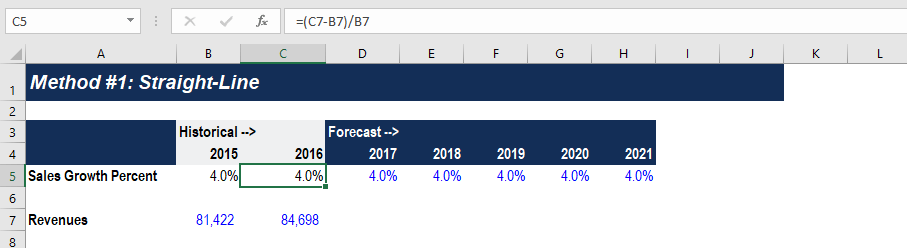2. To forecast future revenues, take the previous year’s figure and multiply it by the growth rate. The formula used to calculate 2017 revenue is =C7*(1+D5).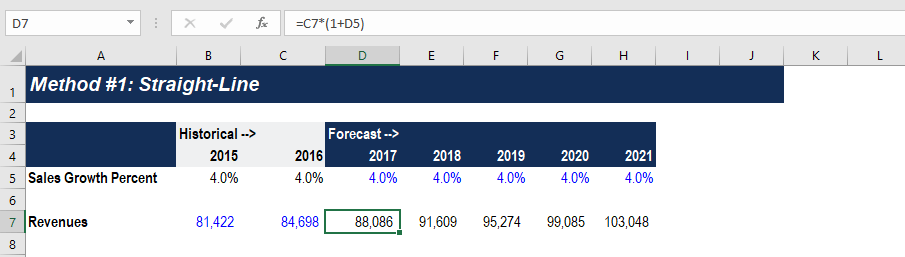3. Select cell D7 to H7, then use the shortcut Ctrl + R to copy the formula all the way to the right.

### #2 Moving Average

Moving averages is a smoothing technique that looks at the underlying pattern of a set of data to establish an estimate of future values. The most common types are the 3-month and 5-month moving averages.

1. To perform a moving average forecast, the revenue data should be placed in the vertical column. Create two columns for 3-month moving averages and 5-month moving averages.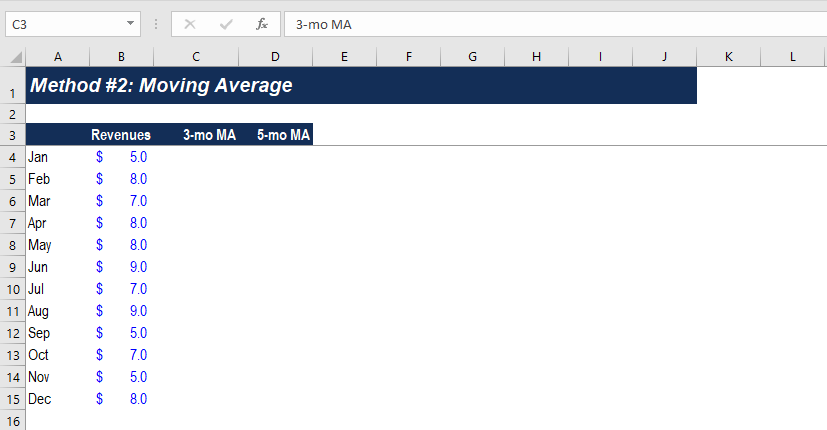2. The 3-month moving average is calculated by taking the average of the current and past two months revenues. The first forecast should begin in March, which is cell C6. The formula used is =AVERAGE(B4:B6), which calculates the average revenue from January to March. Use Ctrl + D to copy the formula down through December.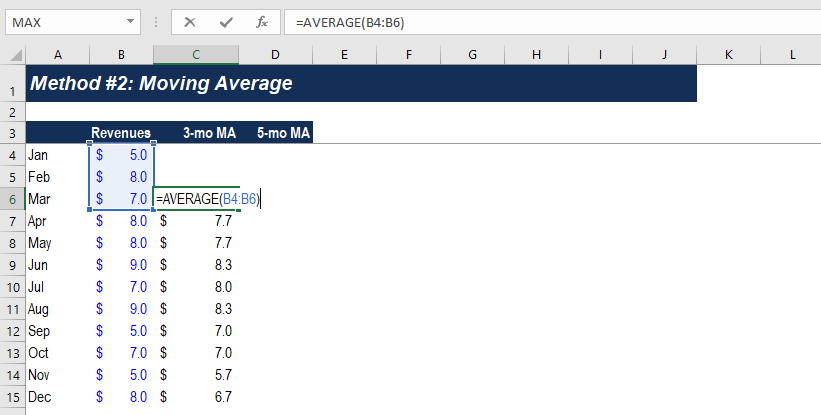3. Similarly, the 5-month moving average forecasts revenue starting the fifth period, which is May. In cell D8, we use the formula =AVERAGE(B4:B8) to calculate the average revenue for January to May. Copy the formula down using shortcut Ctrl + D.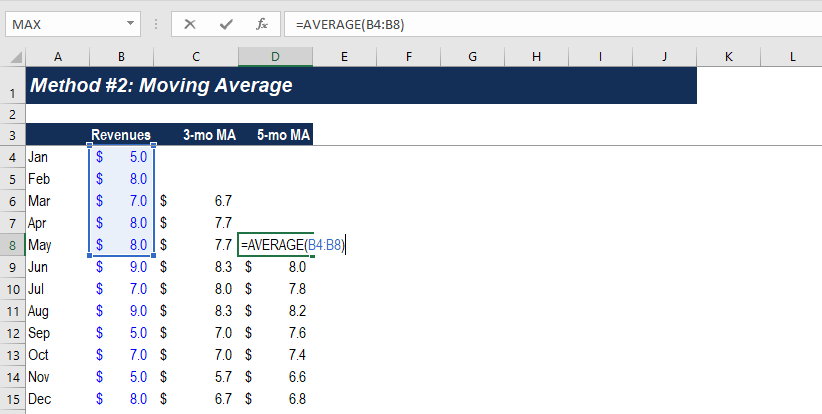4. It is always a good idea to create a line chart to show the difference between actual and MA forecasted values in revenue forecasting methods. Notice that the 3-month MA varies in a greater degree with a significant increase or decrease in historic revenues compared to the 5-month MA. When deciding the time period for a moving average technique, an analyst should consider whether the forecasts should be more reflective of reality or they should smooth out recent fluctuations.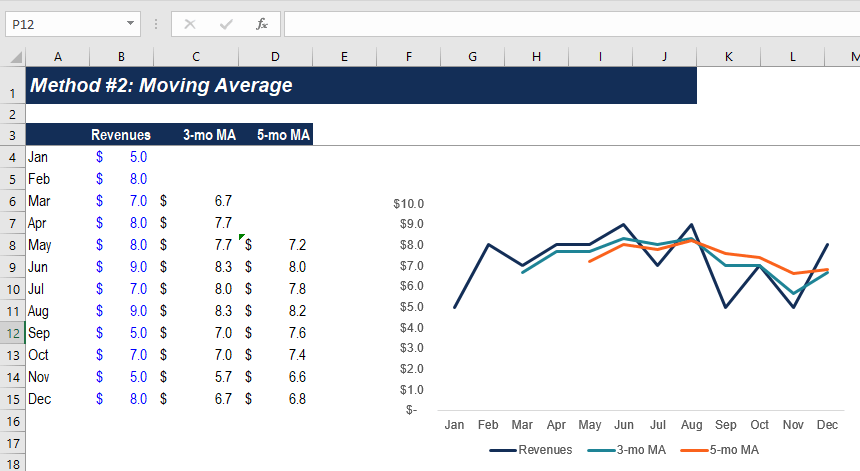### #3 Simple Linear Regression

Regression analysis is a widely used tool for analyzing the relationship between variables for prediction purposes. In another example of revenue forecasting methods here, we will look at the relationship between radio ads and revenue by running a regression on the two variables.

1. Select the Radio ads and Revenue data in cell B4 to C15, then go to Insert > Chart > Scatter.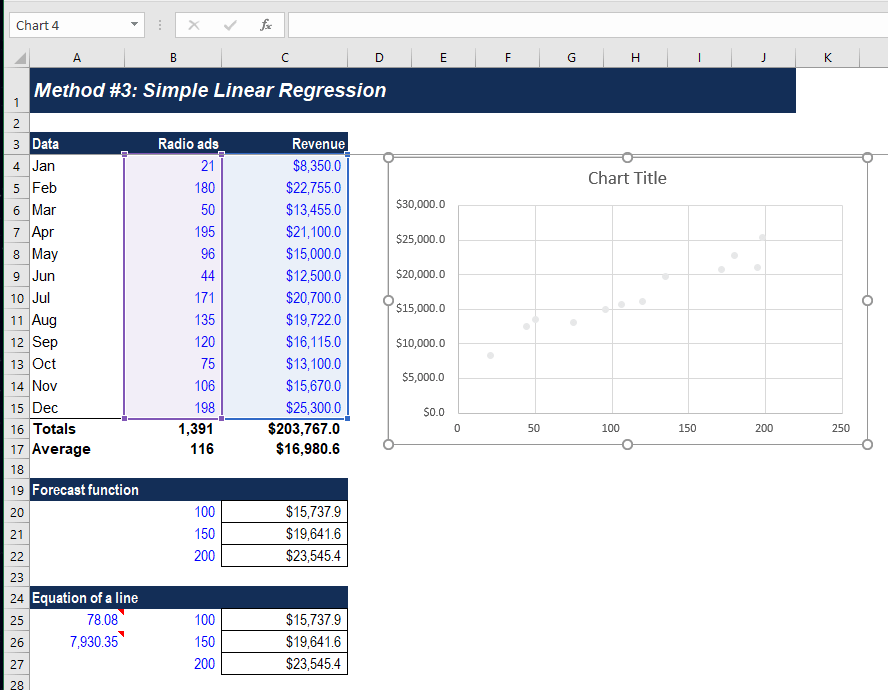2. Right-click on the data points and select Format Data Series. Under Market Options, change color to desired and choose no borderline.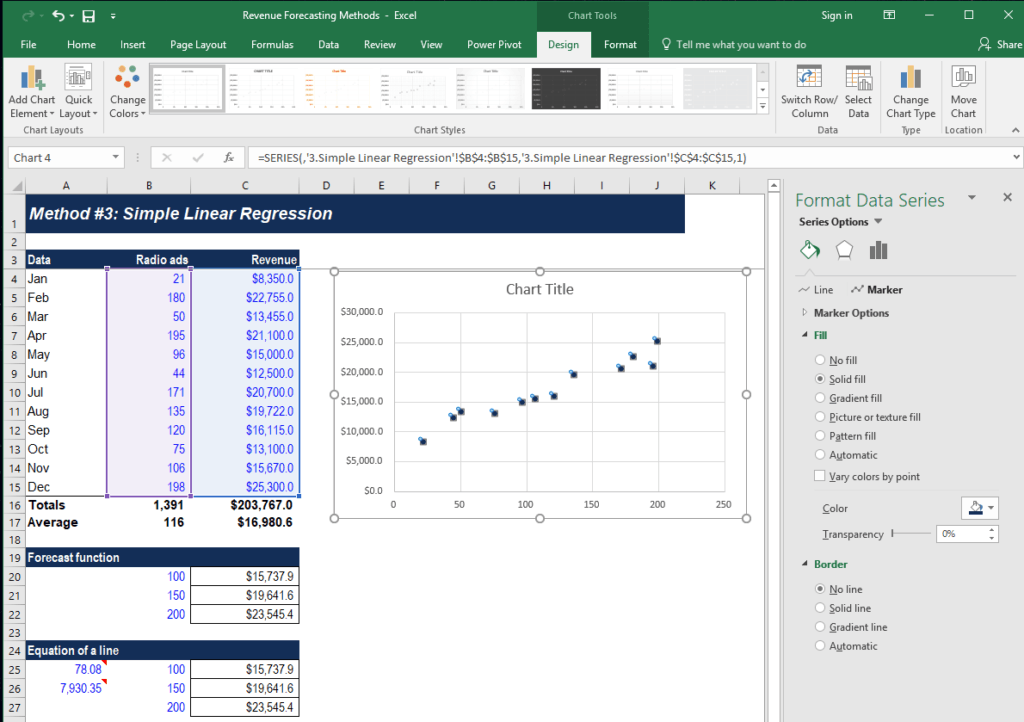3. Right-click on data points and select Add Trendline. Choose Linear line and check the boxes for Display Equation on the chart and Display R-squared value on the chart. Move the equation box to below the line. Increase line width to 3 pt to make it more visible.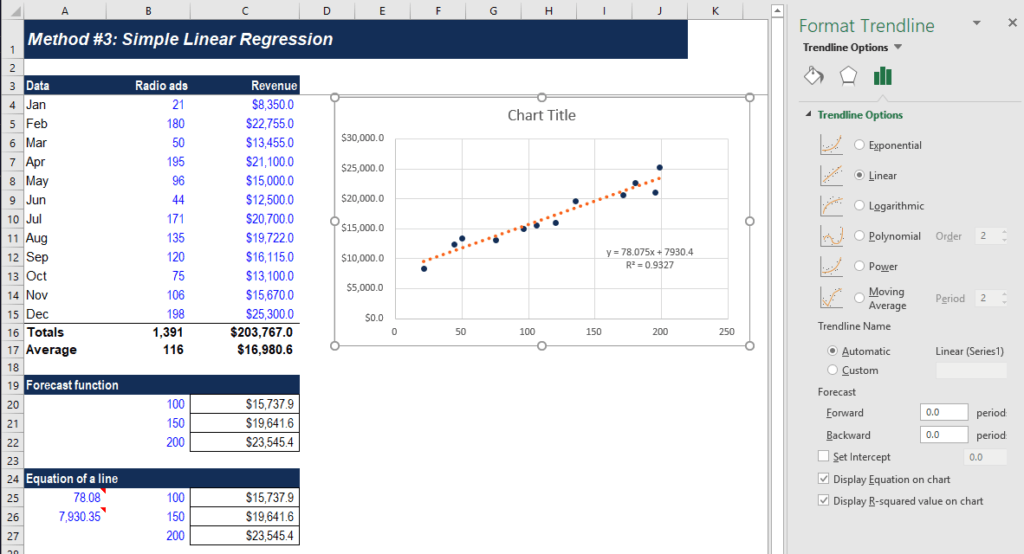4. Choose no fill and no borderline for both chart area and plot area. Remove vertical and horizontal grid lines in the chart.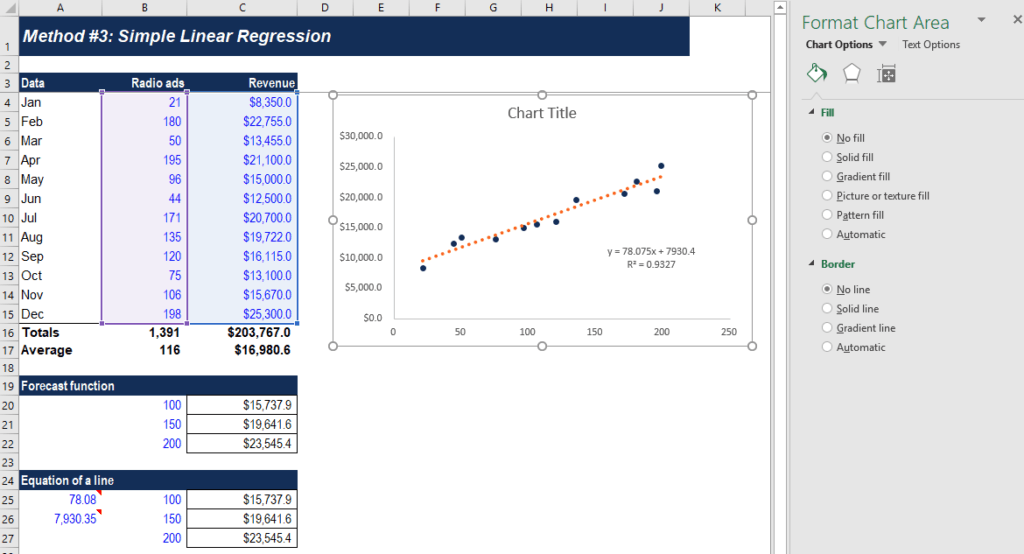5. In the Design ribbon, go to Add Chart Element and insert both horizontal and vertical axis titles. Rename vertical axis to “Revenue” and horizontal axis to “Number of radio ads.” Change chart title to “Relationship between ads and revenue.”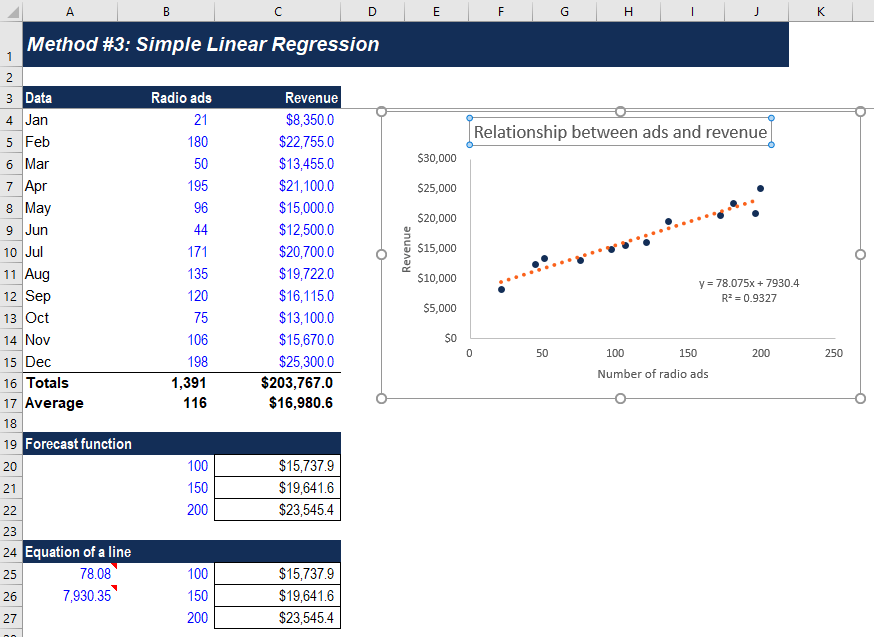6. Besides creating a linear regression line, you can also forecast the revenue using the forecast function in Excel. For example, the company releases 100 ads in the next month and wants to forecast its revenue based on regression. In cell C20, use the formula = FORECAST(B20,\$C\$4:\$C\$15,\$B\$4:\$B\$15). The formula takes data from the Radio ads and Revenue columns to generate a forecast.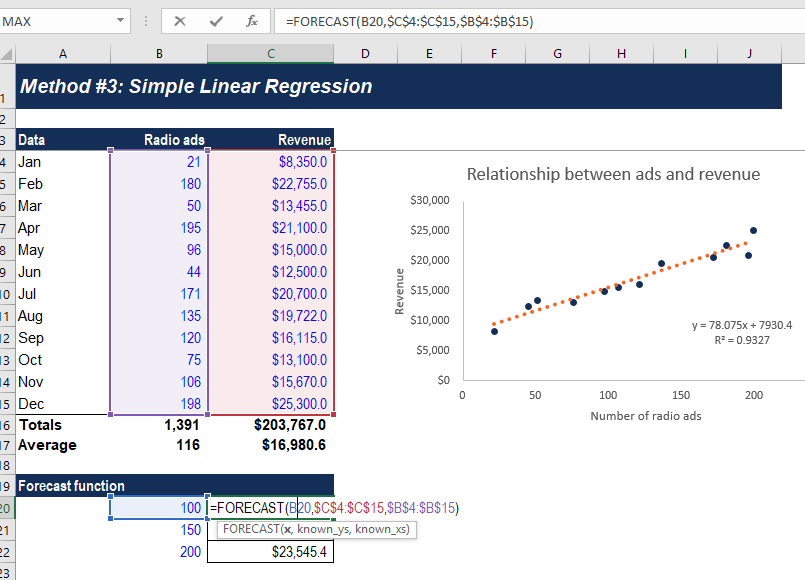7. Another method is to use the equation of the regression line. The slope of the line is 78.08 and the y-intercept is 7930.35. We can use these two numbers to calculate forecasted revenue based on certain x value. In cell C25, we can use the formula =(\$A\$25*B25)+\$A\$26 to find out revenue if there are 100 radio ads.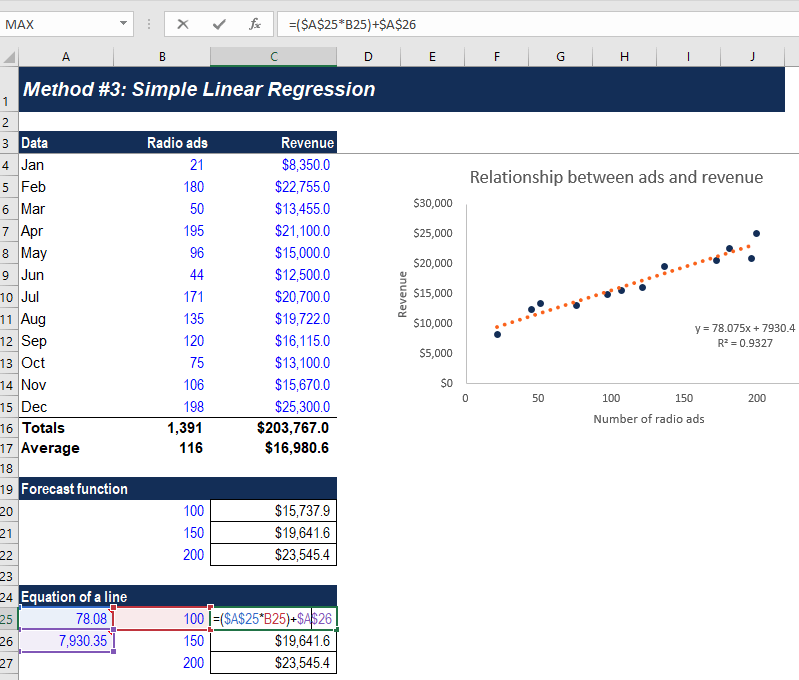### #4 Multiple Linear Regression

A company uses multiple linear regression to forecast revenues when two or more independent variables are required for a projection. In the example below, we will run a regression on promotion cost, advertising cost, and revenue to identify the relationships between these variables.

1. Go to Data tab > Data Analysis > Regression. Select D3 to D15 for Input Y Range and B3 to C15 for Input X Range. Check the box for Labels. Set Output Range at cell A33.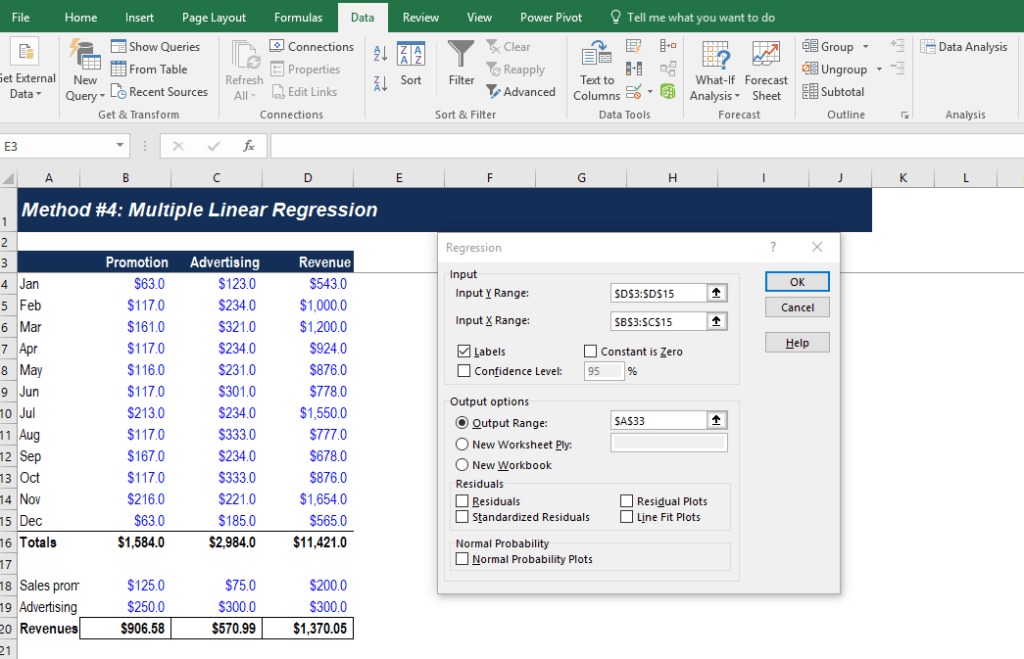2. Copy the very last table from the summary output and paste it in cell A24. Using the coefficients from the table, we can forecast the revenue given the promotion cost and advertising cost. For example, if we expect the promotion cost to be 125 and advertising cost to be 250, we can use the equation in cell B20 to forecast revenue: =\$B\$25+(B18*\$B\$26)+(B19*\$B\$27).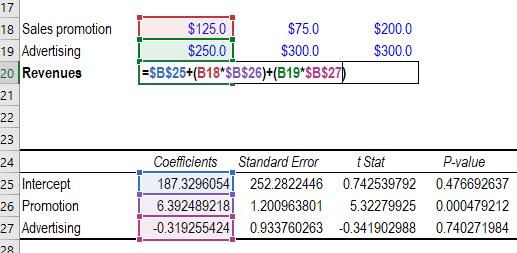### More Resources

Thank you for reading this guide to the top forecasting methods. CFI is the official provider of the global Financial Modeling & Valuation Analyst (FMVA)™ certification program, designed to help anyone become a world-class financial analyst. To keep advancing your career, the additional resources below will be useful:

• Guide to Financial Modeling
• 3 Statement Model
• Forecasting Income Statement Line Items
• Forecasting Balance Sheet Line Items

### Financial Modeling Certification

Become a certified Financial Modeling and Valuation Analyst (FMVA)® by completing CFI’s online financial modeling classes!